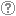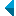# Search the repositoryQuery: search in TitleAuthorAbstractKeywordsFull textYear of publishing ANDORAND NOT search in TitleAuthorAbstractKeywordsFull textYear of publishing ANDORAND NOT search in TitleAuthorAbstractKeywordsFull textYear of publishing ANDORAND NOT search in TitleAuthorAbstractKeywordsFull textYear of publishing Work type: All work types Habilitation (m4) Specialist thesis (m3) High school thesis (m6) Bachelor work * (dip) Master disertations * (mag) Doctorate disertations * (dok) Research Data or Corpuses (data) * old and bologna study programme Language: All languagesSlovenianEnglishGermanCroatianSerbianBosnianBulgarianCzechFinnishFrenchGerman (Austria)HungarianItalianJapaneseLithuanianNorwegianPolishRussianSerbian (cyrillic)SlovakSpanishSwedishTurkishUnknown Search in: RUP    FAMNIT - Faculty of Mathematics, Science and Information Technologies    FHŠ - Faculty of Humanities    FM - Faculty of Management    FTŠ Turistica - Turistica – College of Tourism Portorož    FVZ - Faculty of Health Sciences    IAM - Andrej Marušič Institute    PEF - Faculty of Education    UPR - University of Primorska    ZUP - University of Primorska PressCOBISS    Fakulteta za humanistične študije, Koper    Fakulteta za management Koper in Pedagoška fakulteta Koper    Fakulteta za vede o zdravju, Izola    Knjižnica za tehniko, medicino in naravoslovje, Koper    Turistica, Portorož    Znanstveno-raziskovalno središče Koper Options: Show only hits with full text Reset

 1 - 10 / 4012341.On bipartite Q-polynomial distance-regular graphs with c [sub] 2 [equal] 1Štefko Miklavič, 2007, original scientific articleAbstract: Let ▫$\Gamma$▫ denote a bipartite ▫$Q$▫-polynomial distance-regular graph with diameter ▫$d \ge 3$▫, valency ▫$k \ge 3$▫ and intersection number ▫$c_2=1$▫. We show that ▫$\Gamma$▫ has a certain equitable partition of its vertex set which involves ▫$4d-4$▫ cells. We use this partition to show that the intersection numbers of ▫$\Gamma$▫ satisfy the following divisibility conditions: (I) ▫$c_{i+1}-1$▫ divides ▫$c_i(c_i-1)$▫ for ▫$2 \le i \le d-1$▫, and (II) ▫$b_{i-1}-1$▫ divides ▫$b_i(b_i-1)$▫ for ▫$1 \le i \le d-1$▫. Using these divisibility conditions we show that ▫$\Gamma$▫ does not exist if ▫$d=4$▫.Found in: ključnih besedahSummary of found: ...mathematics, grah theory, distance-regular graphs, ▫$Q$▫-polynomial property, equitable... Keywords: mathematics, grah theory, distance-regular graphs, ▫$Q$▫-polynomial property, equitable partitionsPublished: 15.10.2013; Views: 3262; Downloads: 35Full text (0,00 KB) 2.Isomorphism checking of I-graphsTomaž Pisanski, Boris Horvat, Arjana Žitnik, 2012, original scientific articleAbstract: We consider the class of ▫$I$▫-graphs, which is a generalization of the class of the generalized Petersen graphs. We show that two ▫$I$▫-graphs ▫$I(n, j, k)$▫ and ▫$I(n, j_1, k_1)$▫ are isomorphic if and only if there exists an integer ▫$a$▫ relatively prime to $n$ such that either ▫$\{j_1, k_1\} = \{aj \mod n, \; ak \mod n \}$▫ or ▫$\{j_1, k_1\} = \{aj \mod n, \; -ak \mod n\}$▫. This result has an application in the enumeration of non-isomorphic ▫$I$▫-graphs and unit-distance representations of generalized Petersen graphs.Found in: ključnih besedahSummary of found: ...mathematics, graph theory, isomorphism, I-graph, generalized Petersen graph... Keywords: mathematics, graph theory, isomorphism, I-graph, generalized Petersen graphPublished: 15.10.2013; Views: 3116; Downloads: 130Full text (0,00 KB) 3.Rank-permutable additive mappingsAleksandr Èmilevič Guterman, Anna A. Alieva, Bojan Kuzma, 2006, original scientific articleAbstract: Let ▫$\sigma$▫ be a fixed non-identical permutation on ▫$k$▫ elements. Additive bijections ▫$T$▫ on the matrix algebra ▫$M_n(\mathbb{F})$▫ over a field ▫$\mathbb{F}$▫ of characteristic zero, with the property that ▫$\rm{rk} (A_1...A_k) = \rm{rk} (A_{\sigma(1)}...A_{\sigma(k)})$▫ implies the same condition on the ▫$T$▫ images, are characterized. It is also shown that the surjectivity assumption can be relaxed, if this property is preserved in both directions.Found in: ključnih besedahSummary of found: ...mathematics, linearna algebra, matrix algebra, rank, permutation, additive... Keywords: mathematics, linearna algebra, matrix algebra, rank, permutation, additive preserversPublished: 15.10.2013; Views: 2631; Downloads: 85Full text (0,00 KB) 4.Identities with generalized skew derivations on Lie idealsAjda Fošner, Vincenzo De Filippis, Feng Wei, 2013, original scientific articleAbstract: Let ▫$m, n$▫ be two nonzero fixed positive integers, ▫$R$▫ a 2-torsion free prime ring with the right Martindale quotient ring ▫$Q$▫, ▫$L$▫ a non-central Lie ideal of ▫$R$▫, and ▫$\delta$▫ a derivation of ▫$R$▫. Suppose that ▫$\alpha$▫ is an automorphism of ▫$R$▫, ▫$D$▫ a skew derivation of ▫$R$▫ with the associated automorphism ▫$\alpha$▫, and ▫$F$▫ a generalized skew derivation of ▫$R$▫ with the associated skew derivation ▫$D$▫. If ▫$$F(x^{m+n}) = F(x^m)x^n + x^m \delta (x^n)$$▫ is a polynomial identity for ▫$L$▫, then either ▫$R$▫ satisfies the standard polynomial identity ▫$s_4(x_1, x_2, x_3, x_4)$▫ of degree 4, or ▫$F$▫ is a generalized derivation of ▫$R$▫ and ▫$\delta = D$▫. Furthermore, in the latter case one of the following statements holds: (1) ▫$D = \delta = 0$▫ and there exists ▫$a \in Q$▫ such that ▫$F(x) = ax$▫ for all ▫$x \in R$▫; (2) ▫$\alpha$▫ is the identical mapping of ▫$R$▫.Found in: ključnih besedahSummary of found: ...mathematics, algebra, polynomial identity, generalized skew derivation, prime... Keywords: mathematics, algebra, polynomial identity, generalized skew derivation, prime ringPublished: 15.10.2013; Views: 3138; Downloads: 138Full text (0,00 KB) 5.Q-polynomial distance-regular graphs with a [sub] 1 [equal] 0 and a [sub] 2 [not equal] 0Štefko Miklavič, 2008, original scientific articleAbstract: Let ▫$\Gamma$▫ denote a ▫$Q$▫-polynomial distance-regular graph with diameter ▫$D \ge 3$▫ and intersection numbers ▫$a_1=0$▫, ▫$a_2 \ne 0$▫. Let ▫$X$▫ denote the vertex set of ▫$\Gamma$▫ and let ▫$A \in {\mathrm{Mat}}_X ({\mathbb{C}})$▫ denote the adjacency matrix of ▫$\Gamma$▫. Fix ▫$x \in X$▫ and let denote $A^\ast \in {\mathrm{Mat}}_X ({\mathbb{C}})$ the corresponding dual adjacency matrix. Let ▫$T$▫ denote the subalgebra of ▫$A{\mathrm{Mat}}_X ({\mathbb{C}})$▫ generated by ▫$A$▫, ▫$A^\ast$▫. We call ▫$T$▫ the Terwilliger algebra of ▫$\Gamma$▫ with respect to ▫$x$▫. We show that up to isomorphism there exists a unique irreducible ▫$T$▫-module ▫$W$▫ with endpoint 1. We show that ▫$W$▫ has dimension ▫$2D-2$▫. We display a basis for ▫$W$▫ which consists of eigenvectors for ▫$A^\ast$▫. We display the action of ▫$A$▫ on this basis. We show that ▫$W$▫ appears in the standard module of ▫$\Gamma$▫ with multiplicity ▫$k-1$▫, where ▫$k$▫ is the valency of ▫$\Gamma$▫.Found in: ključnih besedahSummary of found: ...mathematics, graph theory, adjacency matrix, distance-regular graph, Terwilliger... Keywords: mathematics, graph theory, adjacency matrix, distance-regular graph, Terwilliger algebraPublished: 15.10.2013; Views: 3251; Downloads: 27Full text (0,00 KB) 6.On quartic half-arc-transitive metacirculantsDragan Marušič, Primož Šparl, 2008, original scientific articleAbstract: Following Alspach and Parsons, a metacirculant graph is a graph admitting a transitive group generated by two automorphisms ▫$\rho$▫ and ▫$\sigma$▫, where ▫$\rho$▫ is ▫$(m,n)$▫-semiregular for some integers ▫$m \ge 1$▫, ▫$n \ge 2▫$, and where ▫$\sigma$▫ normalizes ▫$\rho$▫, cyclically permuting the orbits of ▫$\rho$▫ in such a way that ▫$\sigma^m$▫ has at least one fixed vertex. A half-arc-transitive graph is a vertex- and edge- but not arc-transitive graph. In this article quartic half-arc-transitive metacirculants are explored and their connection to the so called tightly attached quartic half-arc-transitive graphs is explored. It is shown that there are three essentially different possibilities for a quartic half-arc-transitive metacirculant which is not tightly attached to exist. These graphs are extensively studied and some infinite families of such graphs are constructed.Found in: ključnih besedahSummary of found: ...mathematics, graph theory, metacirculant graph, half-arc-transitive graph, tightly... Keywords: mathematics, graph theory, metacirculant graph, half-arc-transitive graph, tightly attached, automorphism groupPublished: 15.10.2013; Views: 3032; Downloads: 125Full text (0,00 KB) 7.Consistent Cycles in 1/2-Arc-Transitive GraphsŠtefko Miklavič, Marko Boben, Primož Potočnik, 2009, original scientific articleFound in: ključnih besedahSummary of found: ...mathematics, graph theory, 1/2-arc-transitivity, consistent cycle, ... Keywords: mathematics, graph theory, 1/2-arc-transitivity, consistent cyclePublished: 15.10.2013; Views: 3504; Downloads: 35Full text (0,00 KB)This document has more files! More... 8.Leonard triples and hypercubesŠtefko Miklavič, 2007, original scientific articleAbstract: Let ▫$V$▫ denote a vector space over ▫$\mathbb{C}$▫ with finite positive dimension. By a Leonard triple on ▫$V$▫ we mean an ordered triple of linear operators on ▫$V$▫ such that for each of these operators there exists a basis of ▫$V$▫ with respect to which the matrix representing that operator is diagonal and the matrices representing the other two operators are irreducible tridiagonal. Let ▫$D$▫ denote a positive integer and let ▫${\mathcal{Q}}_D$▫ denote the graph of the ▫$D$▫-dimensional hypercube. Let ▫$X$ denote the vertex set of ▫${\mathcal{Q}}_D$▫ and let ▫$A \in {\mathrm{Mat}}_X ({\mathbb{C}})$▫ denote the adjacency matrix of ▫${\mathcal{Q}}_D$▫. Fix ▫$x \in X$▫ and let ▫$A^\ast \in {\mathrm{Mat}}_X({\mathbb{C}})$▫ denote the corresponding dual adjacency matrix. Let ▫$T$▫ denote the subalgebra of ▫${\mathrm{Mat}}_X({\mathbb{C}})$ generated by ▫$A,A^\ast$▫. We refer to ▫$T$▫ as the Terwilliger algebra of ▫${\mathcal{Q}}_D$▫ with respect to ▫$x$▫. The matrices ▫$A$▫ and ▫$A^\ast$▫ are related by the fact that ▫$2iA = A^\ast A^\varepsilon - A^\varepsilon A^\ast$▫ and ▫$2iA^\ast = A^\varepsilon A - AA^\varepsilon$▫, where ▫$2iA^\varepsilon = AA^\ast - A^\ast A$▫ and ▫$i^2 = -1$▫. We show that the triple ▫$A$▫, ▫$A^\ast$▫, ▫$A^\varepsilon$▫ acts on each irreducible ▫$T$▫-module as a Leonard triple. We give a detailed description of these Leonard triples.Found in: ključnih besedahSummary of found: ...mathematics, graph theory, Leonard triple, distance-regular graph, hypercube,... Keywords: mathematics, graph theory, Leonard triple, distance-regular graph, hypercube, Terwilliger algebraPublished: 15.10.2013; Views: 2922; Downloads: 115Full text (0,00 KB) 9.On generalized Jordan triple ([alpha], [beta]) [sup] [ast]-derivations and related mappingsAjda Fošner, Shakir Ali, Maja Fošner, Mohammad Salahuddin Khan, 2013, original scientific articleAbstract: Let ▫$R$▫ be a 2-torsion free semiprime ▫$\ast$▫-ring and let ▫$\alpha, \beta$▫ be surjective endomorphisms of ▫$R$▫. The aim of the paper is to show that every generalized Jordan triple ▫$(\alpha, \beta)^\ast$▫-derivation on ▫$R$▫ is a generalized Jordan ▫$(\alpha, \beta)^\ast$▫-derivation. This result makes it possible to prove that every generalized Jordan triple ▫$(\alpha, \beta)^\ast$▫-derivation on a semisimple ▫$H^\ast$▫-algebra is a generalized Jordan ▫$(\alpha, \beta)^\ast$▫-derivation. Finally, we prove that every Jordan triple left ▫$\alpha^\ast$▫-centralizer on a 2-torsion free semiprime ring is a Jordan left ▫$\alpha^\ast$▫-centralizer.Found in: ključnih besedahSummary of found: ...mathematics, algebra, semiprime ▫$\ast$▫-ring, ▫$H^\ast$▫-algebra, Jordan triple ▫$(\alpha,... Keywords: mathematics, algebra, semiprime ▫$\ast$▫-ring, ▫$H^\ast$▫-algebra, Jordan triple ▫$(\alpha, \beta)^\ast$▫-derivation, generalized Jordan triple ▫$(\alpha, \beta)^\ast$▫-derivation, Jordan triple left ▫$\alpha^\ast$▫-centralizerPublished: 15.10.2013; Views: 2824; Downloads: 69Full text (0,00 KB) 10.Semiovals contained in the union of three concurrent linesIstván Kovács, György Kiss, Aart Blokhuis, Aleksander Malnič, Dragan Marušič, János Ruff, 2007, original scientific articleAbstract: Semiovals which are contained in the union of three concurrent lines are studied. The notion of a strong semioval is introduced, and a complete classification of these objects in PG▫$(2,p)$▫ and PG▫$(2,p^2)$▫, ▫$p\$▫ an odd prime, is given.Found in: ključnih besedahSummary of found: ...mathematics, semioval, group factorization... Keywords: mathematics, semioval, group factorizationPublished: 15.10.2013; Views: 2291; Downloads: 124Full text (0,00 KB)
Search done in 0 sec.